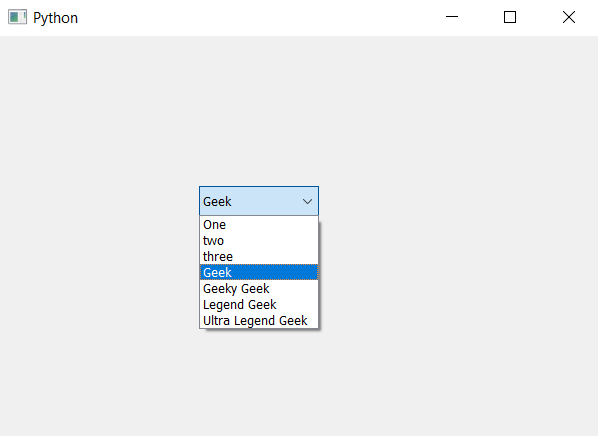# PyQt5 – Adding multiple items at given index in ComboBox

• Last Updated : 18 Jan, 2022

In this article we will see how we can add multiple items in the combo box at the given index. We know we can add item with the help of addItem and addItems method but items get added at the end only, in order to add the multiple items at the given index we use insertItems method.

Syntax : combo_box.insertItems(index, item_list)
Argument : It takes two argument one is integer i.e index and second is list i.e list with each element is string
Action performed : It will add items at the given index

Below is the implementation –

## Python3

 `# importing libraries``from` `PyQt5.QtWidgets ``import` `*``from` `PyQt5 ``import` `QtCore, QtGui``from` `PyQt5.QtGui ``import` `*``from` `PyQt5.QtCore ``import` `*``import` `sys`  `class` `Window(QMainWindow):` `    ``def` `__init__(``self``):``        ``super``().__init__()` `        ``# setting title``        ``self``.setWindowTitle("Python ")` `        ``# setting geometry``        ``self``.setGeometry(``100``, ``100``, ``600``, ``400``)` `        ``# calling method``        ``self``.UiComponents()` `        ``# showing all the widgets``        ``self``.show()` `    ``# method for widgets``    ``def` `UiComponents(``self``):` `        ``# creating a combo box widget``        ``self``.combo_box ``=` `QComboBox(``self``)` `        ``# setting geometry of combo box``        ``self``.combo_box.setGeometry(``200``, ``150``, ``120``, ``30``)` `        ``# geek list``        ``geek_list ``=` `["Geek", "Geeky Geek", "Legend Geek", "Ultra Legend Geek"]` `        ``# adding list of items to combo box``        ``self``.combo_box.addItems(geek_list)` `        ``# new item list``        ``new ``=` `["One", "two", "three"]``        ` `        ``# adding item list at index 0``        ``self``.combo_box.insertItems(``0``, new)` `# create pyqt5 app``App ``=` `QApplication(sys.argv)` `# create the instance of our Window``window ``=` `Window()` `# start the app``sys.exit(App.``exec``())`

Output :My Personal Notes arrow_drop_up